# Corrections

Equations A.11 and A.12 required a conventional notation correction for second derivatives.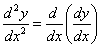(A.11)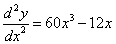(A.12)

The following equations had summation symbols removed to reflect the fact that, when taking a partial derivative of a function with respect to a certain variable, all the terms in the summation other than those containing that variable are regarded as constant, and consequently vanish (A.14).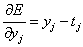(2.7)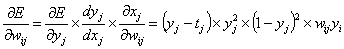(2.18)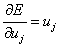(B.5)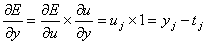(B.7)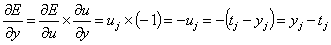(B.11)

Thanks to Martin Jimenez, Yoshio Takane, and Denis Mareschal for pointing out mathematical problems and/or suggesting corrections.